# Assertion & Reason Test: Polynomials - 2

## 10 Questions MCQ Test Online MCQ Tests for Class 10 | Assertion & Reason Test: Polynomials - 2

Description
Attempt Assertion & Reason Test: Polynomials - 2 | 10 questions in 20 minutes | Mock test for Class 10 preparation | Free important questions MCQ to study Online MCQ Tests for Class 10 for Class 10 Exam | Download free PDF with solutions
QUESTION: 1

### Direction: In the following questions, a statement of assertion (A) is followed by a statement of reason (R). Mark the correct choice as:Assertion : x2 + 4x + 5 has two zeroes.Reason : A quadratic polynomial can have at the most two zeroes.

Solution: p(x) = 0 ⇒ x2 + 4x + 5 = 0

Discriminant, D = b2 – 4ac

= 42 – 4 x 1 x 5

= 16 – 20 = – 4 < 0

Therefore, no real zeroes are there.

QUESTION: 2

### Direction: In the following questions, a statement of assertion (A) is followed by a statement of reason (R). Mark the correct choice as:Assertion (A): If the zeroes of the quadratic polynomial (k – 1) x2 + kx + 1 is – 3, then the value of k is 4/3.Reason (R): If – 1 is a zero of the polynomial p(x) = kx2 – 4x + k, then the value of k is –2.

Solution:

In case of assertion:

Let p(x) = (k – 1)x2 + kx + 1

As –3 is a zero of p(x), then p (−3) = 0

⇒ (k – 1) (−3)2 + k (−3) + 1 = 0

⇒ 9k – 9 – 3k + 1 = 0

⇒ 9k – 3k = +9 – 1

⇒ 6k = 8

⇒ k = 4/3

∴ Given statement is correct.

In case of reason:

Since, – 1 is a zero of the polynomial

and p(x) = kx2 – 4x + k, then p (–1) = 0

∴ k (–1)2 – 4 (–1) + k = 0

⇒ k + 4 + k = 0

⇒ 2k + 4 = 0

⇒ 2k = – 4

Hence, k = – 2

∴ Given statement is correct.

Thus, both assertion and reason are correct but reason is not the correct explanation for assertion.

QUESTION: 3

### Direction: In the following questions, a statement of assertion (A) is followed by a statement of reason (R). Mark the correct choice as:Assertion : If one zero of poly-nominal p(x) = (k2 + 4)x2 + 13x + 4k is reciprocal of other, then k = 2.Reason : If (x - α) is a factor of p(x), then p(α) = 0i.e. α is a zero of p(x).

Solution: Reason is true.

Let α, 1/α be the zeroes of p(x), then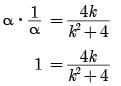k2 - 4k + 4 = 0

(k - 2)2 = 0

k = 2 Assertion is true Since, Reason is not correct for Assertion.

QUESTION: 4

Direction: In the following questions, a statement of assertion (A) is followed by a statement of reason (R). Mark the correct choice as:

Assertion : The graph y = f(x) is shown in figure, for the polynomial f(x). The number of zeros of f(x) is 3.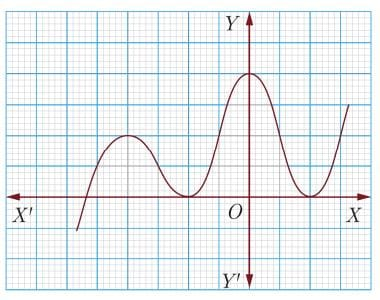Reason : The number of zero of the polynomial f(x) is the number of point of which f(x) cuts or touches the axes.

Solution:

As the number of zeroes of polynomial f(x) is the number of points at which f(x) cuts (intersects) the x –axis and number of zero in the given figure is 3.

So A is correct but R is not correct.

QUESTION: 5

Direction: In the following questions, a statement of assertion (A) is followed by a statement of reason (R). Mark the correct choice as:

Assertion : P(x) = 14x3 - 2x2 + 8x4 + 7x - 8 is a polynomial of degree 3.

Reason : The highest power of x in the polynomial p(x) is the degree of the polynomial.

Solution: The highest power of x in the polynomial p(x)

= 14x3 - 2x2 + 8x4 + 7x - 8x is 4.

Degree of p(x) is 4. So, A is incorrect but R is correct.

QUESTION: 6

Direction: In the following questions, a statement of assertion (A) is followed by a statement of reason (R). Mark the correct choice as:

Assertion : The graph y = f(x) is shown in figure, for the polynomial f (x). The number of zeros of f(x) is 3.

Reason : The number of zero of the polynomial f(x) is the number of point of which f(x) cuts or touches the axes.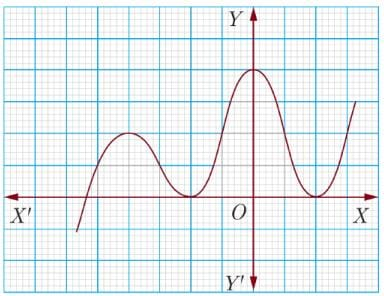Solution: As the number of zeroes of polynomial f(x) is the number of points at which f(x) cuts (intersects) the x –axis and number of zero in the given figure is 3.

So A is correct but R is not correct.

QUESTION: 7

Direction: In the following questions, a statement of assertion (A) is followed by a statement of reason (R). Mark the correct choice as:

Assertion : x3 + x has only one real zero.

Reason : A polynomial of nth degree must have n real zeroes.

Solution: Reason is false [a polynomial of nth degree has at most x zeroes.]

Again, x3 + x = x(x2 + 1)

which has only one real zero (x = 0)

[x2 + 1 ≠ 0 for all x ε R]

Assertion is true.

QUESTION: 8

Direction: In the following questions, a statement of assertion (A) is followed by a statement of reason (R). Mark the correct choice as:

Assertion: Degree of a zero polynomial is not defined.

Reason: Degree of a non-zero constant polynomial is 0

Solution: We know that, the constant polynomial 0 is called a zero polynomial. The degree of a zero polynomial is not defined.

So, Assertion is true. Now, the degree of a non-zero constant polynomial is zero. So, Reason is true.

QUESTION: 9

Direction: In the following questions, a statement of assertion (A) is followed by a statement of reason (R). Mark the correct choice as:

Assertion: The sum and product of the zeros of a quadratic polynomial are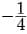and 1/4 respectively.

Then the quadratic polynomial is 4x2 + x + 1.

Reason : The quadratic polynomial whose sum and product of zeros are given is x2-(sum of zeros). x + product of zeros.

Solution: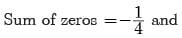product of zeros = 1/4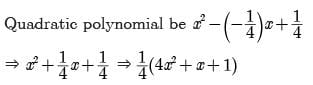Quadratic polynomial be 4x2 + x + 1. So, both A and R are correct and R explains A.

QUESTION: 10

Direction: In the following questions, a statement of assertion (A) is followed by a statement of reason (R). Mark the correct choice as:

Assertion : x2 + 7x + 12 has no real zeroes.

Reason : A quadratic polynomial can have at the most two zeroes.

Solution: x2 + 7x + 12 = 0

⇒ x2 + 4x + 3x + 12 = 0

⇒ x(x + 4) + 3(x + 4) = 0

⇒ (x + 4) (x + 3) = 0

⇒ (x + 4) = 0 or (x + 3) = 0

⇒ x = −4 or x = −3

Therefore, x2 + 7x + 12 has two real zeroes.Use Code STAYHOME200 and get INR 200 additional OFF Use Coupon Code